Next: Scale Factor Evolution Up: Field Equations and Coordinate Previous: Field Equations

## Selecting the Rescaling Variables

The program allows the user to set the rescaling variables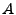,,, and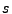to whatever is most useful for a particular model (up to one caveat discussed below). However there are certain guidelines that we suggest following in setting these variables. These guidelines are written into the program as defaults in the file model.h. (See the section on how to implement new models for more details on how the program sets these variables.) These default settings are based on the following criteria:

1. We want to eliminate the first derivative term from the equation of motion. This will make the equations simpler to solve.
2. We want to set the scale of the field variables to be of order unity, at least initially. This will make numerical calculations simpler as well as making the output more readable.
3. We want the coefficient of the dominant potential term to be of order unity. This means the program time variable will automatically measure the natural time scale of the problem.
4. We want the coefficient of the dominant potential term to include no powers of the scale factor. If this weren't true the time scale of the calculations would be changing as the program progressed and a fixed time step would be untenable.
The first two of these conditions immediately give us two equations(6.7)

and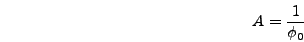(6.8)

whereis the initial value of the inflaton (or whatever field dominates initially). The latter two conditions require that we assume some form for the dominant potential term. We assume that term to be polynomial; if you wish to consider a model where the dominant term is not polynomial you should expand it in a Taylor series and will hopefully be able to identify an effective dominant polynomial term. So take this dominant term to be of the form(6.9)

Then the corresponding term that appears in the equation of motion forwill be(6.10)

so the last two criteria above give(6.11)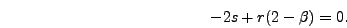(6.12)

Putting all these equations together(6.13)

If you choose to use these defaults you simply have to set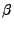,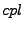, andfor your model and the other variables will be set automatically according to equation (6.13). Otherwise you can directly change the definitions of,,, and. All of these definitions are in the file model.h. If you do explicitly change the four rescaling parameters you should set the initial field values and derivatives as needed for your rescaling scheme. (These values are all set in the file parameters.h.) However, the relationship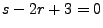must be maintained because the evolution equations don't include a first derivative term and will thus be invalid if this relationship is violated. The variable definitions in model.h explicitly set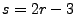so you can independently set,, andto whatever you wish.

Using these default values the field equation becomes(6.14)

where(6.15)

Note that this expression for the potential will pick up additional rescaling factors whenis expressed in terms of the program fields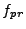. For example a quartic coupling of the form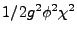will give rise to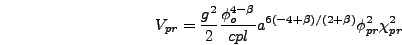(6.16)(6.17)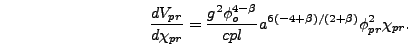(6.18)Next: Scale Factor Evolution Up: Field Equations and Coordinate Previous: Field Equations